# Characteristic function

(diff) ← Older revision | Latest revision (diff) | Newer revision → (diff)

Fourier–Stieltjes transform, of a probability measureThe complex-valued function given on the entire axis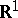by the formulaThe characteristic function of a random variableis, by definition, that of its probability distribution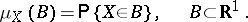A method connected with the use of characteristic functions was first applied by A.M. Lyapunov and later became one of the basic analytical methods in probability theory. It is used most effectively in proving limit theorems of probability theory. For example, the proof of the central limit theorem for independent identically-distributed random variables with second moments reduces to the elementary relation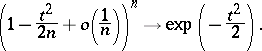## Contents

### Basic properties of characteristic functions.

1)and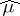is positive definite, i.e.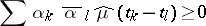for any finite sets of complex numbers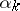and arguments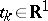;

2)is uniformly continuous on the entire axis;

3),,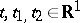.

4)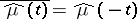; in particular,takes only real values (and is an even function) if and only if the corresponding probability distribution is symmetric, i.e., where.

5) The characteristic function determines the measure uniquely; the inversion formula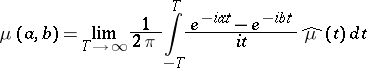is valid for any interval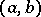for which the end pointsare continuity points of. Ifis integrable (absolutely if the integral is understood in the sense of Riemann) on, then the corresponding distribution function has a densityand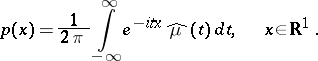6) The characteristic function of the convolution of two probability measures (of the sum of two independent random variables) is the product of their characteristic functions.

The following three properties express the connection between the existence of moments of a random variable and the order of smoothness of its characteristic function.

7) If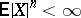for some natural number, then for all natural numbersthe derivative of orderof the characteristic function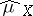of the random variableexists and satisfies the equationHence,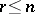.

8) If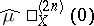exists, then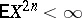;

9) Iffor alland if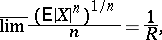then for all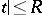,The use of the method of characteristic functions is based mainly on the properties of characteristic functions indicated above and also on the following two theorems.

Bochner's theorem (description of the class of characteristic functions). Suppose that a functionis given onand that. Forto be the characteristic function of some probability measure it is necessary and sufficient that it be continuous and positive definite.

Lévy's theorem (continuity of the correspondence). Letbe a sequence of probability measures and let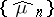be the sequence of their characteristic functions. Then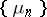weakly converges to some probability measure(that is,for an arbitrary continuous bounded function) if and only ifconverges at every pointto some continuous function; in the case of convergence,. This implies that the relative compactness (in the sense of weak convergence) of a family of probability measures is equivalent to the equicontinuity at zero of the family of corresponding characteristic functions.

Bochner's theorem makes it possible to view the Fourier–Stieltjes transform as an isomorphism between the semi-group (under the operation of convolution) of probability measures onand the semi-group (under pointwise multiplication) of positive-definite continuous functions onthat have at zero the value one. Lévy's theorem asserts that this algebraic isomorphism is also a topological homeomorphism if in the semi-group of probability measures one has in mind the topology of weak convergence, and in the semi-group of positive-definite functions the topology of uniform convergence on bounded sets.

Expressions are known for the characteristic functions of the basic probability measures (see , ). For example, the characteristic function of the Gaussian measure with meanand variance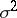is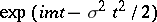.

For non-negative integer-valued random variablesone uses, apart from the characteristic function, also its analogue: the generating function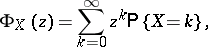which is connected with the characteristic function by the relation.

The characteristic function of a probability measureon a finite-dimensional space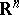is defined similarly: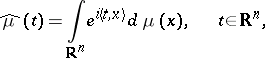where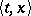denotes the scalar product. The facts stated above are also valid for characteristic functions of probability measures on.

How to Cite This Entry:
Characteristic function. Encyclopedia of Mathematics. URL: http://encyclopediaofmath.org/index.php?title=Characteristic_function&oldid=15699
This article was adapted from an original article by N.N. Vakhania (originator), which appeared in Encyclopedia of Mathematics - ISBN 1402006098. See original article